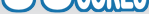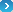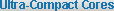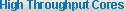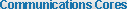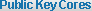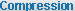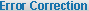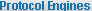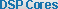Elliptic Curve Point Multiply and Verify CoreGeneral DescriptionElliptic Curve Cryptography (ECC) is a public-key cryptographic technology that uses the mathematics of so called “elliptic curves” and it is a part of the “Suite B” of cryptographic algorithms approved by the NSA.Since ECC requires fewer bits than RSA to achieve the same cipher strength, it is frequently used in embedded applications. The operations necessary for the ECC cannot be efficiently implemented on an embedded CPU, however, typically requiring hundreds of milliseconds of the CPU time for signature verification.ECC1 implements by far the most time-consuming operation of the ECC cryptography: so called “point multiplication” to enable low-power operation of the battery-powered devices. It also supports the “point verification” operation to simplify the system integration.The design is fully synchronous and available in multiple configurations varying in bus widths, set of elliptic curves supported and throughput.Key FeaturesECC1 implementation is unencumbered by any patentsSmall size: ECC1 requires less than 10K ASIC gatesHigh throughput for long life battery powered applications: 5,000 point multiplications per second in the 65 nm ASIC processSupport for the NIST ECC binary fields 2163, 2233, 2283, 2409, and 2571Microprocessor-friendly interfaceTest bench providedSymbol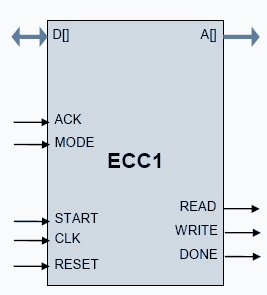ApplicationsSecure communications systemsRFIDImplantable medical devicesDigital Rights Management (DRM) for battery powered electronicsElliptic Curve Diffie-Hellman (EC-DH) standard ANSI X9.63Elliptic Curve Digital Signature Algorithm (EC-DSA) standard ANSI X9.62Digital Signature Standard (DSS) FIPS-186B and K elliptic curves (163, 233, 283, 409, 571) defined by NISTIEEE P1363 curves over binary fields GF(2m)TLS implementations per RFC 4492Cryptographic messaging per RFC 3278Zigbee SE ProfilePin Description

 Name Type Description CLK Input Core clock signal START Input When HIGH, starts a Point Multiply or Point Verify operation RESET Input HIGH level asynchronously resets the core READ Output Read request for the memory WRITE Output Write request for the memory DONE Output HIGH level indicates a completion of computation D[] Input memory data bus A[] Input Address for the memory ACK Input Red/write acknowledgement from the memory MODE Input LOW level for Point Multiply, HIGH for Point Verify operationElliptic Curve Cryptography Basics

Elliptic curve cryptography (ECC) is an approach to public-key cryptography based on the algebraic structure of elliptic curves over finite fields. The use of elliptic curves in cryptography was suggested independently by Neal Koblitz and Victor S. Miller in 1985.

A binary field is a finite field GF(2m) which contains 2m elements for some m (called the degree of the field). The elements of this field are the bit strings of length m, and the field arithmetic is implemented in terms of operations on the bits.

An elliptic curve is a plane curve which is isomorphic to a curve defined by an equation of the form
y2 = x3 + ax + b

The set of points on such a curve — all solutions of the above equation together with a point at infinity — form an Abelian group, with the point at infinity as identity element and a generator element G. The use of elliptic curves in cryptography is based on an assumption that the “point multiplication” operation (for an integer k, finding kG = G+G+G+…+G) is relatively easy to calculate, but very hard to “reverse” (i.e., finding k when only kG and G are known requires enormous computing power for large k and m).

The two critical ECC operations that greatly benefit from hardware acceleration are:“Point multiplication”, i.e. calculation of coordinates of the point Q = kP given an integer k and point P. This operation is by far the most computationally intensive, and its hardware implementation improves the performance of the ECC algorithm and power consumed by the device.“Point verification” simply verifies if the given point P indeed belongs to the curve. This is important, because the value of kP for a point P that does not belong to the curve, might reveal the secret random number k. Although the verification is much less computationally intensive than the multiplication, its implementation in hardware allows to provide a complete solution (no finite field calculations in firmware) at virtually zero cost in gates.

The following table gives the approximate size (m) of the binary filed used in ECC to match the corresponding strength of the symmetric cipher (per NIST recommendations). For comparison, the bit length of comparable RSA public key solutions is also provided.

 Symmetric key bit length Matching binary ECC bit length m Matching RSA bit length 80 163 1024 112 (3DES) 233 2048 128 (AES-128) 283 3072 256 (AES-256) 571 15360

For each field degree m, NIST defined a pseudo-random curve along with a Koblitz curve. The pseudo-random curve has the form

y 2 + x y = x 3 + x 2 + b,

and the Koblitz curve has the form Ea:

y2 + x y = x 3 + ax 2 + 1

where a = 0 or 1.Function Description

The core implements the point multiplication operation and the point verification operations. The operands for the multiplication: k, Px, Py are placed into the shared memory before the start of operation. Once the operation is complete, the results are placed back into the shared memory. The curve parameters a/b/m as well as the width of CPU memory are fixed for the particular version of the core, for example the ECC1-163/32 core work with the NIST binary curve of 163 bits and has a 32-bit wide interface to the CPU memory.

Design of ECC1 allows sharing the arbitrated CPU memory to store the arguments and results of operations. This both helps to save the silicon resource in the extremely compact implementations (smart cards) and simplify the data transfer between the CPU and the ECC1 core. The memory load during the core operation is very small, about 5% of the total cycles. If sharing memory is not desirable, an optional dedicated memory can be used.Implementation Details

Representative synthesis results for the ECC1-163/32 are shown below.

 Technology Max Frequency Area/Resources TSMC 65 nm 835 MHz 9,972 gates TSMC 65 nm 1636 MHz 14,109 gates TSMC 90 nm 1064 MHz 11,399 gates Altera MAX II 139 MHz 2059 LE Altera Cyclone II 220 MHz 2065 LE Altera Stratix II 250 MHz 1137 ALUTs Xilinx Virtex 5 265 MHz 630 slices Actel ProASIC3 48 MHz 7790 tilesExport Permits
The core is subject to the US export regulations. See the IP Cores, Inc. licensing basics page,
http://ipcores.com/export_licensing.htm, for links to US government sites and licensing details.DeliverablesSynthesizable Verilog RTL source codeSoftware modules for a complete ECC implementation (optional)Verilog testbench (self-checking)Vectors for testbenchExpected resultsUser DocumentationPost-synthesis EDIFTestbench (self-checking)Vectors for testbenchExpected results